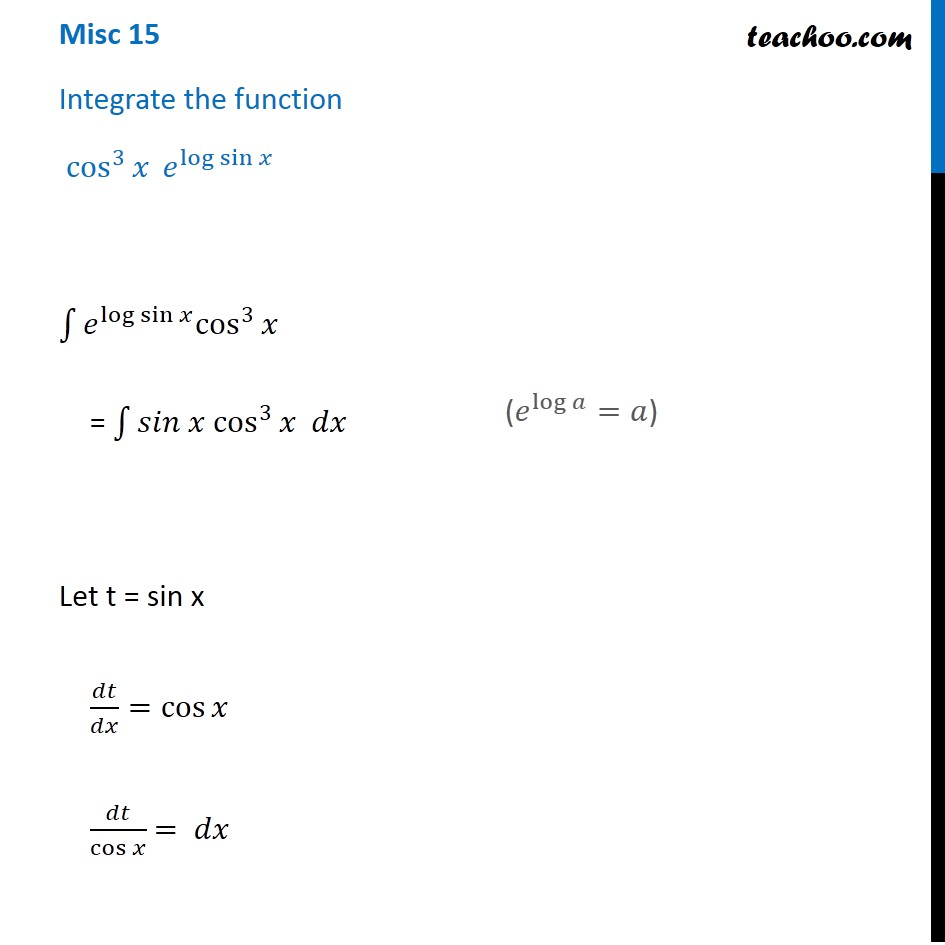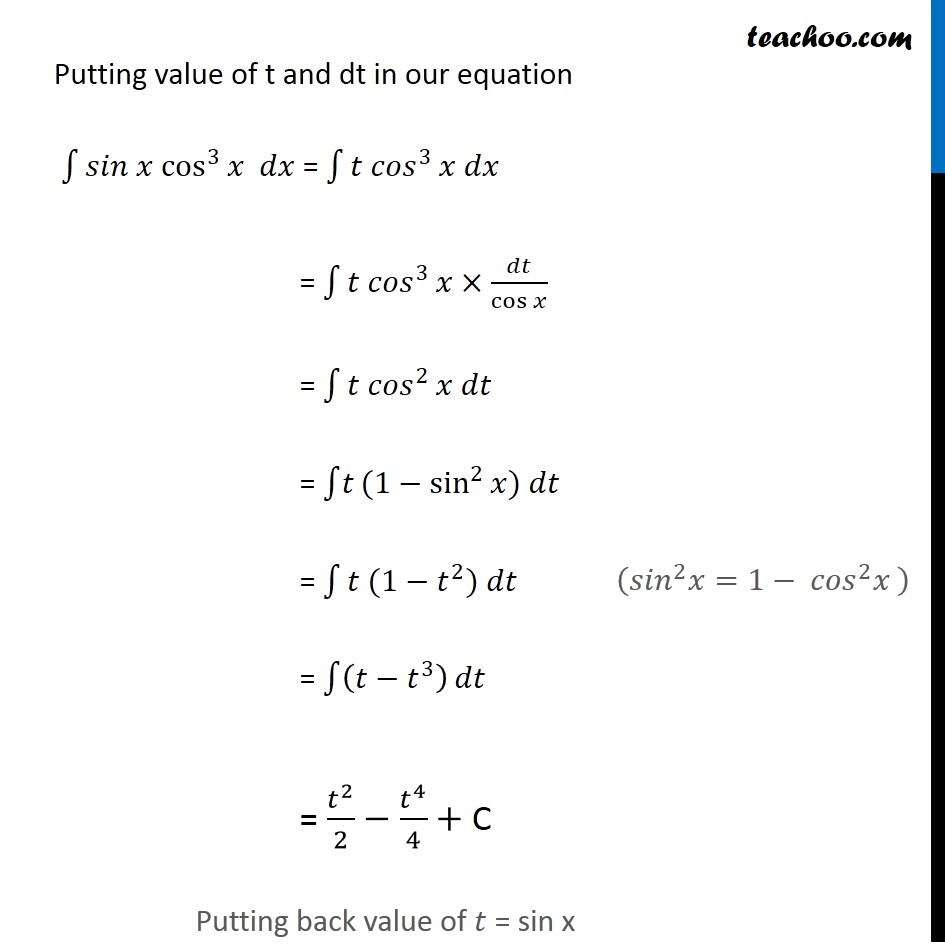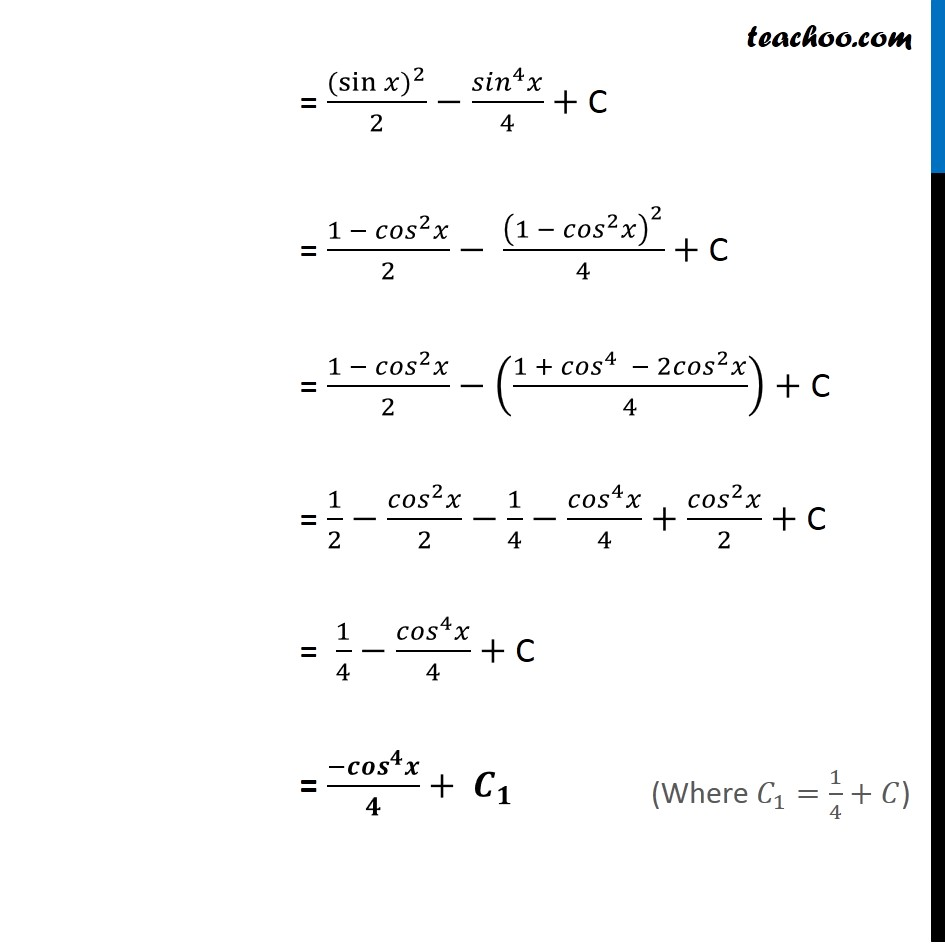Miscellaneous

Chapter 7 Class 12 Integrals
Serial order wiseLearn in your speed, with individual attention - Teachoo Maths 1-on-1 Class

### Transcript

Misc 15 Integrate the function cos^3⁡𝑥 𝑒^log⁡sin⁡𝑥 ∫1▒〖𝑒^log⁡sin⁡𝑥 cos^3〗⁡𝑥 = ∫1▒〖𝑠𝑖𝑛 𝑥 cos^3〗⁡〖𝑥 𝑑𝑥〗 Let t = sin x 𝑑𝑡/𝑑𝑥=cos⁡𝑥 𝑑𝑡/cos⁡𝑥 = 𝑑𝑥 (𝑒^log⁡𝑎 =𝑎) Putting value of t and dt in our equation ∫1▒〖𝑠𝑖𝑛 𝑥 cos^3〗⁡〖𝑥 𝑑𝑥〗 = ∫1▒〖𝑡 〖𝑐𝑜𝑠〗^3 〗 𝑥 𝑑𝑥 = ∫1▒〖𝑡 〖𝑐𝑜𝑠〗^3 〗 𝑥×𝑑𝑡/cos⁡𝑥 = ∫1▒〖𝑡 〖𝑐𝑜𝑠〗^2 〗 𝑥 𝑑𝑡 = ∫1▒𝑡(1−sin^2⁡𝑥) 𝑑𝑡 = ∫1▒〖𝑡 (1−𝑡^2 〗) 𝑑𝑡 = ∫1▒(𝑡−𝑡^3 ) 𝑑𝑡 = 𝑡^2/2−𝑡^4/4+ C Putting back value of 𝑡 = sin x = ((〖sin⁡〖𝑥)〗〗^2)/2−(〖𝑠𝑖𝑛〗^4 𝑥)/4+ C =(1 − 〖𝑐𝑜𝑠〗^2 𝑥)/2− (1 − 〖𝑐𝑜𝑠〗^2 𝑥)^2/4+ C = (1 −〖 𝑐𝑜𝑠〗^2 𝑥)/4−((1 + 〖𝑐𝑜𝑠〗^4 −2〖𝑐𝑜𝑠〗^2 𝑥)/4)+ C = 1/2−(〖𝑐𝑜𝑠〗^2 𝑥)/2−1/4−(〖𝑐𝑜𝑠〗^4 𝑥)/4+(〖𝑐𝑜𝑠〗^2 𝑥)/2+ C = ((〖sin⁡〖𝑥)〗〗^2)/2−(〖𝑠𝑖𝑛〗^4 𝑥)/4+ C = (1 − 〖𝑐𝑜𝑠〗^2 𝑥)/2− (1 − 〖𝑐𝑜𝑠〗^2 𝑥)^2/4+ C = (1 −〖 𝑐𝑜𝑠〗^2 𝑥)/2−((1 + 〖𝑐𝑜𝑠〗^4 − 2〖𝑐𝑜𝑠〗^2 𝑥)/4)+ C = 1/2−(〖𝑐𝑜𝑠〗^2 𝑥)/2−1/4−(〖𝑐𝑜𝑠〗^4 𝑥)/4+(〖𝑐𝑜𝑠〗^2 𝑥)/2+ C = 1/4−(〖𝑐𝑜𝑠〗^4 𝑥)/4+ C = (−〖𝒄𝒐𝒔〗^𝟒 𝒙)/𝟒+ 𝑪_𝟏 (Where 𝐶_1=1/4+𝐶) = ((〖sin⁡〖𝑥)〗〗^2)/2−(〖𝑠𝑖𝑛〗^4 𝑥)/4+ C = (1 − 〖𝑐𝑜𝑠〗^2 𝑥)/2− (1 − 〖𝑐𝑜𝑠〗^2 𝑥)^2/4+ C = (1 −〖 𝑐𝑜𝑠〗^2 𝑥)/2−((1 + 〖𝑐𝑜𝑠〗^4 − 2〖𝑐𝑜𝑠〗^2 𝑥)/4)+ C = 1/2−(〖𝑐𝑜𝑠〗^2 𝑥)/2−1/4−(〖𝑐𝑜𝑠〗^4 𝑥)/4+(〖𝑐𝑜𝑠〗^2 𝑥)/2+ C = 1/4−(〖𝑐𝑜𝑠〗^4 𝑥)/4+ C = (−〖𝒄𝒐𝒔〗^𝟒 𝒙)/𝟒+ 𝑪_𝟏